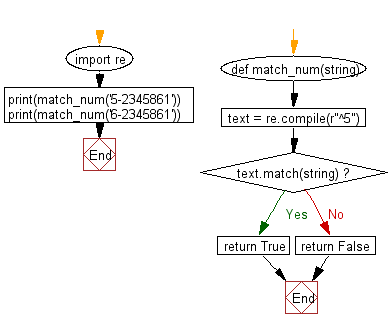﻿ Python: Where a string will start with a specific number - w3resource## Python Regular Expression: Exercise-15 with Solution

Write a Python program where a string will start with a specific number.

Sample Solution:-

Python Code:

``````import re
def match_num(string):
text = re.compile(r"^5")
if text.match(string):
return True
else:
return False
print(match_num('5-2345861'))
print(match_num('6-2345861'))
```
```

Sample Output:

```True
False
```

Pictorial Presentation:Flowchart:## Visualize Python code execution:

The following tool visualize what the computer is doing step-by-step as it executes the said program:

Python Code Editor:

Have another way to solve this solution? Contribute your code (and comments) through Disqus.

What is the difficulty level of this exercise?

Test your Python skills with w3resource's quiz

﻿

## Python: Tips of the Day

Unknown Arguments Using *arguments:

If your function can take in any number of arguments then add a * in front of the parameter name:

```def myfunc(*arguments):
for a in arguments:
print a
myfunc(a)
myfunc(a,b)
myfunc(a,b,c)
```